## 基于ROS搭建简易软件框架实现ROV水下目标跟踪（七）–程序解析之推力分配

139
0
2020年11月5日 09时21分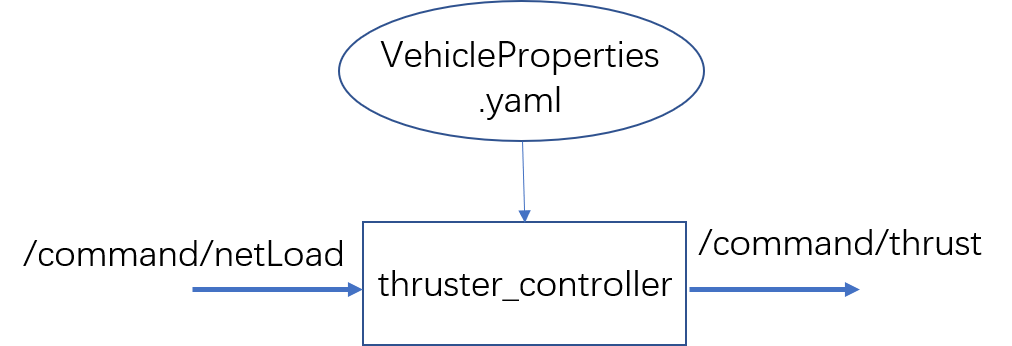Yaml文件为机械模型文件（链接）。

ThrusterController::LoadParam<string>("properties_file", properties_file);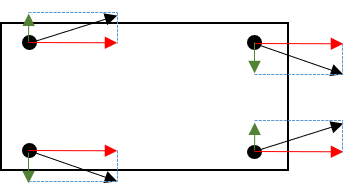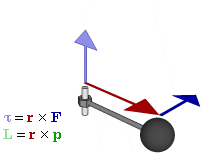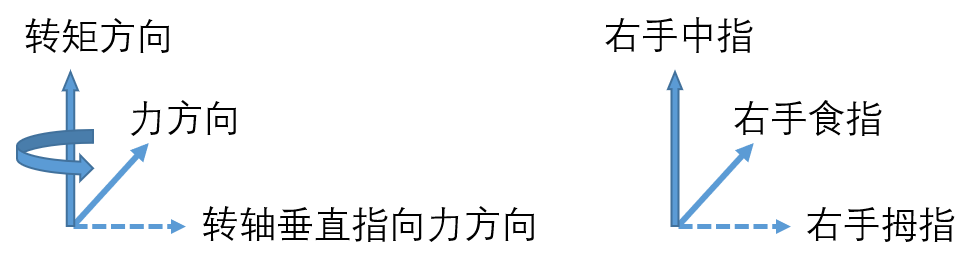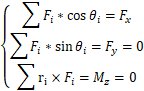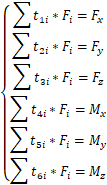for(int i = 0; i < numThrusters; i++){
if(thrustersEnabled[i]){
for(int j = 0; j < 5; j++){
//Transform X, Y, Z to COM reference frame
if(j < 3){
thrusters(j, i) = properties["properties"]["thrusters"][i]["pose"】[j].as<double>() - center_of_mass[j];
}
else{
thrusters(j, i) = properties["properties"]["thrusters"][i]["pose"][j].as<double>();
}
}
}
}


//rotate around z, y axis
float psi = thrusters(3, i) * PI / 180;
float theta = thrusters(4, i) * PI / 180;
thrustCoeffs(0, i) = cos(psi) * cos(theta);  //Effective contribution along X-axis
thrustCoeffs(1, i) = sin(psi) * cos(theta);  //Effective contribution along Y-axis
thrustCoeffs(2, i) = -sin(theta);            //Effective contribution along Z-axis


//cross-product
//Determine the effective moment arms for each thruster about the B-frame axes
thrustCoeffs.block<3, 1>(3, i) = thrusters.block<3, 1>(0, i).cross(thrustCoeffs.block<3, 1>(0, i));


F * thrustCoeffs = [Fx，Fy，Fz，Mx，My，Mz]^T

weightLoad_eig.setZero();
isSubmerged = false;

for(int i = 0; i < 6; i++){
transportThm[i] = 0;
command[i] = 0;
//solver_forces[i] = 0;
}

for(int i = 0; i < 8; i++){
solver_forces[i] = 0;
}

for(int i = 0; i < 3; i++){
solver_cob[i] = 0;
Fb_vector[i] = 0;
}

state_sub = nh.subscribe<cabin_msgs::Imu>("/state/imu", 1, &ThrusterController::ImuCB, this);

ThrusterController::InitThrustMsg();


ThrusterController::InitDynamicReconfigure();


//EOM problem
problemEOM.AddResidualBlock(new ceres::AutoDiffCostFunction<EOM, 6, 8>(new EOM(numThrusters, thrustCoeffs, inertia, weightLoad, transportThm, command)), NULL, solver_forces);
optionsEOM.max_num_iterations = 100;
optionsEOM.linear_solver_type = ceres::DENSE_QR;


//Class EOM defines the 6 equations of motiion that ceres needs to solve
class EOM{
...
}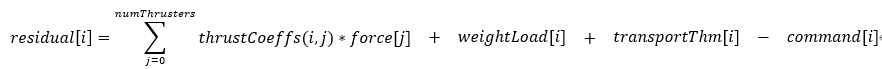1、command为期望的作用于机器人的力与力矩，即[Fx，Fy，Fz，Mx，My，Mz]，理解为输入未尝不可；
2、force为推进器推力；
3、residual为推进器总的推力作用于机器人的力和力矩和期望值的偏差，之后通过最小二乘或其它算法求解推进器推力F；
4、等式左边第一项看过thrustCoeffs的介绍应该能理解；
6、thransportThm程序也是在函数ImuCB中，需要IMU角速度数据及转动惯量，从程序上看是需要抵消掉机器人旋转时的一些影响，我请教了一些机械方向的同事，也没得到答案；
7、对于程序中的inertia这一项，并未参与到运动方程的计算，所以我没将其写入运动方程中。也许俄亥俄州大学最新版本的程序中有对其有进一步的开发吧。
8、solver_forces就是最终计算得到的各推进器推力，就理解为输出吧。

//Buoyancy Problem
problemBuoyancy.AddResidualBlock(new ceres::AutoDiffCostFunction<FindCoB, 3, 3>(new FindCoB(numThrusters, thrustCoeffs, Fb_vector, solver_forces)), NULL, solver_cob);
optionsBuoyancy.max_num_iterations = 100;
optionsBuoyancy.linear_solver_type = ceres::DENSE_QR;


demo中没有用到，程序结构参考EOM problem。